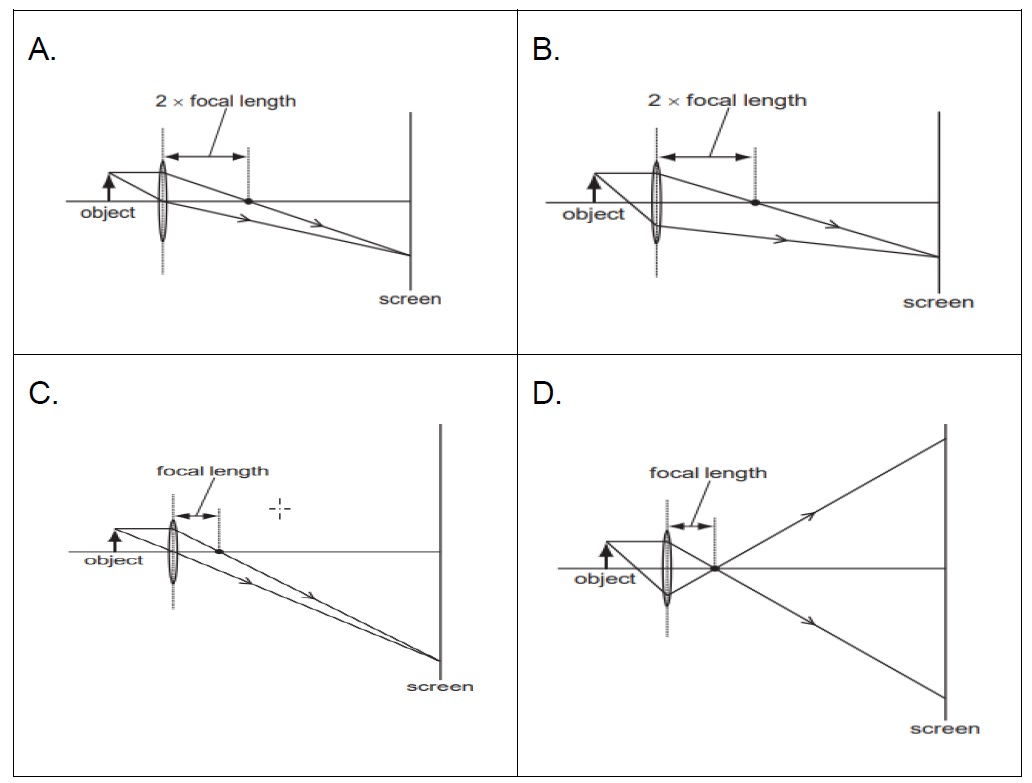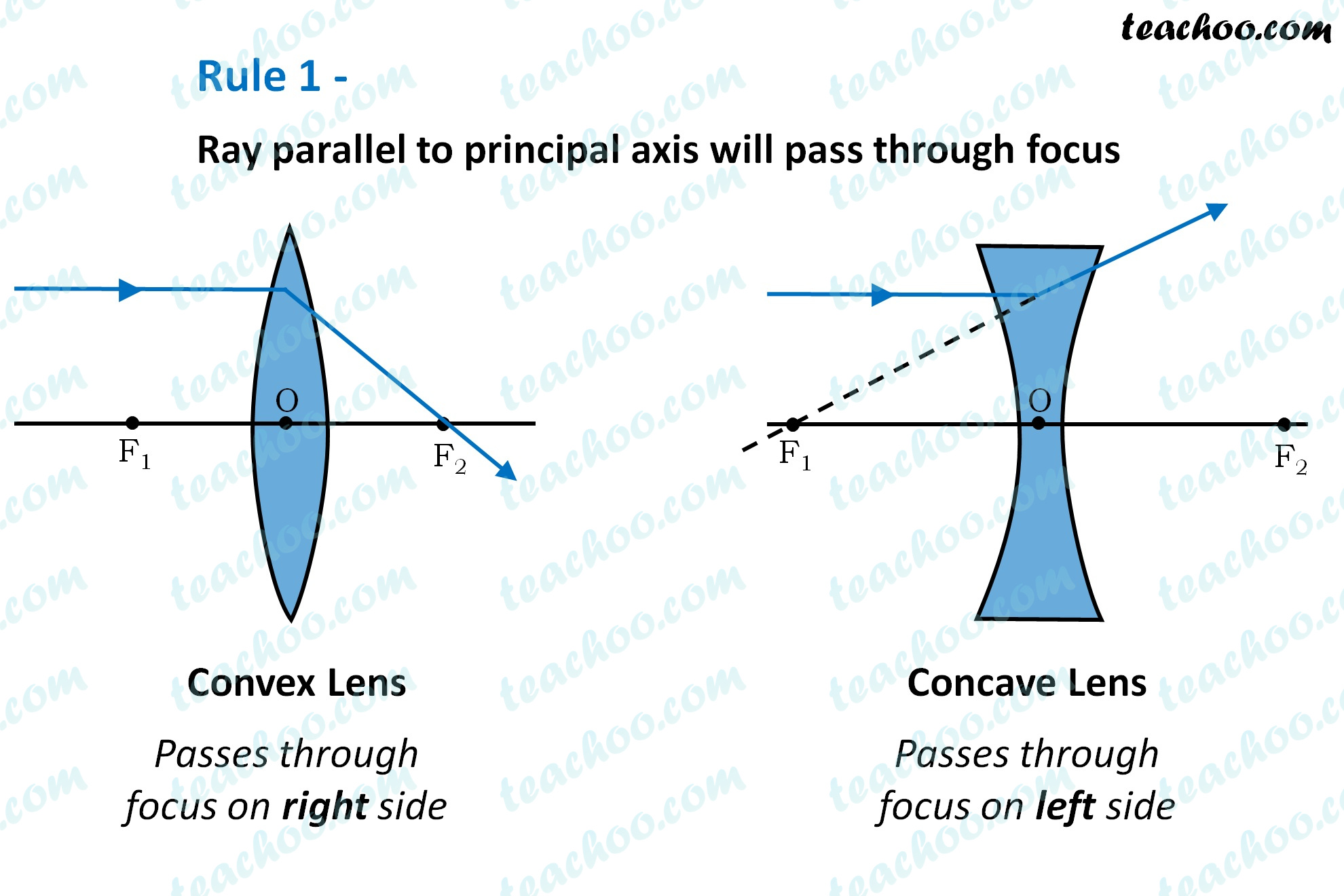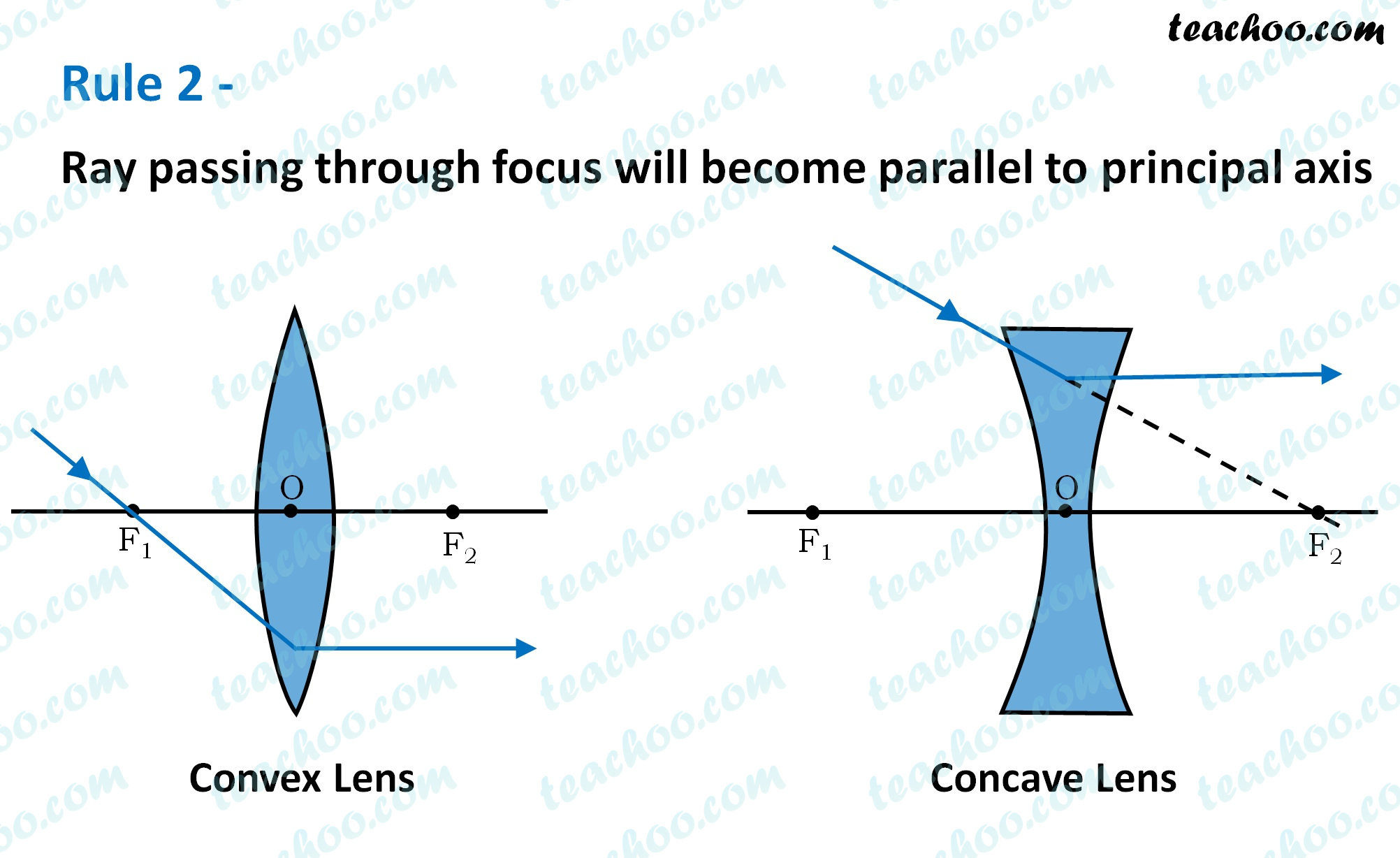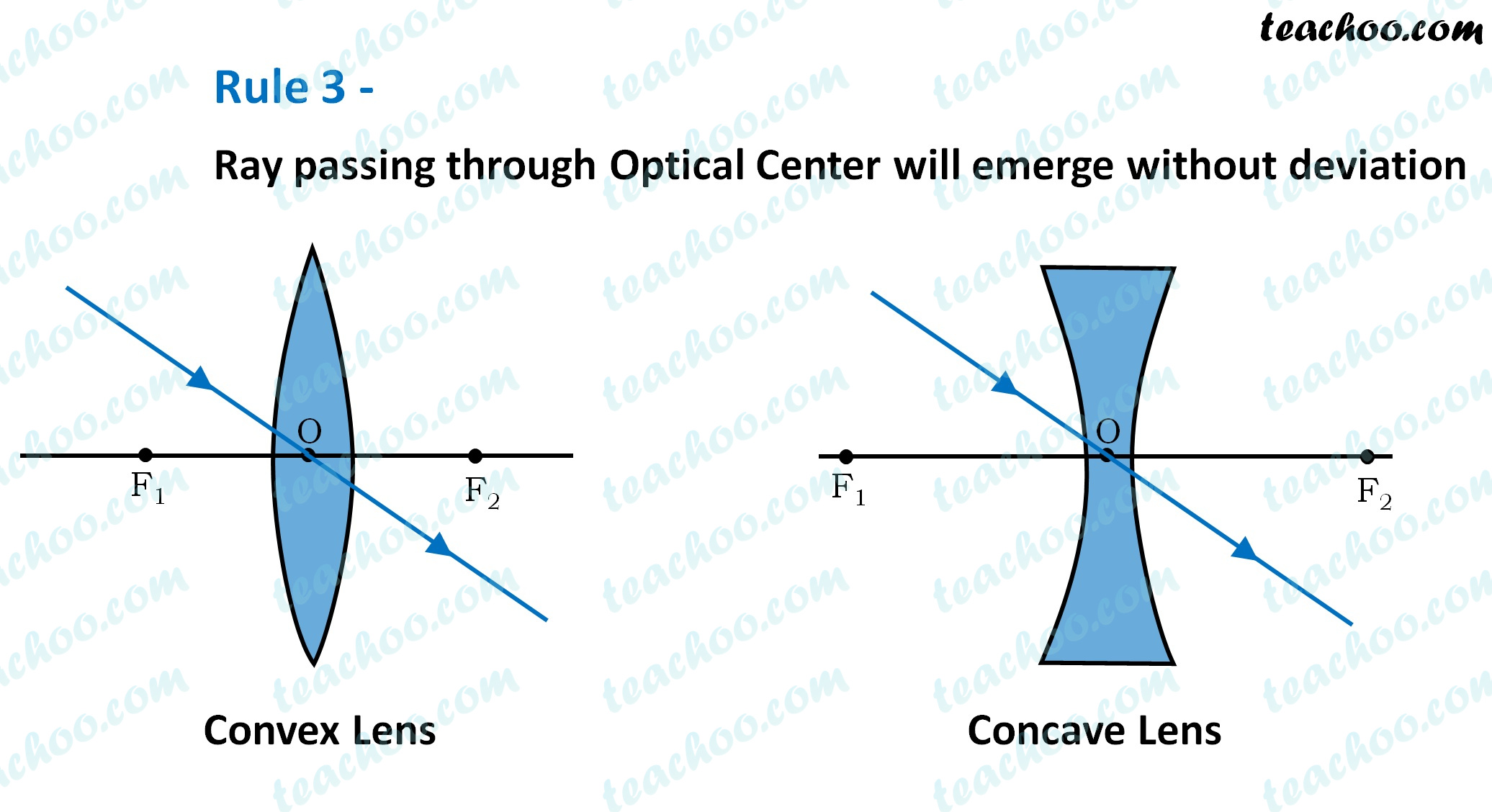Solutions - CBSE Class 10 Sample Paper for 2022 Boards - Science [MCQ]

Class 10
Solutions to CBSE Sample Paper - Science Class 10

## Which diagram shows image formation of an object on a screen by a converging lens?While drawing ray diagram , we follow these rules

• A ray parallel to the principal axis will pass through the principal focus.
• A ray passing through the principal focus will become parallel to the principal axis.
• A ray passing through the optical center will emerge without deviation.In our diagram• In Option A : Converged ray passes through twice focal length, and not focal length. So, this is incorrect.
• In Option B : Converged ray passes through twice focal length , and not focal length. So, this is incorrect.
• In Option C : Converged ray passes through focal length, and ray passing through Optical center does not deviate. Thus, it follows all rules . So, Option C is correct .
• In Option D: Ray passing through focus (bottom ray) will become parallel to principal axis , but here ray passing through focus again converges... which is incorrect. So, this is incorrect .

So, the correct answer is (c)

Get live Maths 1-on-1 Classs - Class 6 to 12